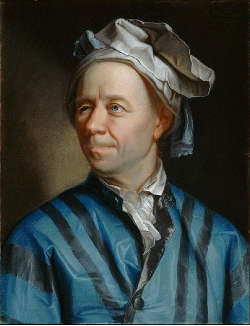### Pi and the Basel's problemIn 1644 the Italian mathematician Pietro Mengoli proposed the so-called Basel's problem, which asked for the exact solution to the square of the sum of the reciprocals of all the natural numbers: $\sum_{n=1}^\infty \frac{1}{n^2} = \frac{1}{1^2} + \frac{1}{2^2} + \cdots$ The solution to the problem came in 1735 thanks to Leonard Euler, at the time at the beginning of his brilliant career as a problem solver. The Swiss mathematician proved that the exact sum of the series is $\pi^2 / 6$.
The Euler's demonstration, published in its final form in 1741, is particularly interesting: Euler supposed that it's possible to apply the rules of the finite polynomials even those endless.
We start with the development in Taylor series for the sine function in 0: $\sin(x) = x - \frac{x^3}{3!} + \frac{x^5}{5!} - \frac{x^7}{7!} + \cdots$ Dividing by $x$ both terms, we obtain: $\frac{\sin(x)}{x} = 1 - \frac{x^2}{3!} + \frac{x^4}{5!} - \frac{x^6}{7!} + \cdots$ whose roots are $\pi$, $-\pi$, $2\pi$, $-2\pi$, $3\pi$, $-3\pi$, $\ldots$ By changing the variable as $z = x^2$, the polynomial above becomes: $\frac{\sin(\sqrt{z})}{\sqrt{z}} = 1 - \frac{z}{3!} + \frac{z^2}{5!} - \frac{z^3}{7!} + \cdots$ whose roots are $\pi^2$, $4\pi^2$, $9\pi^2$, $\ldots$
Now, given a polynomial $a_n x^n + \cdots + a_3 x^3 + a_2 x^2 + bx + 1$, for the formulas of Viète, we have that the sum of the reciprocals of its roots has as result $-b$. Applying this result for finished polynomials to infinite polynomial in $z$ above, we get: $\frac{1}{3!} = \frac{1}{6} = \frac{1}{\pi^2} + \frac{1}{4\pi^2} + \frac{1}{9\pi^2} + \frac{1}{16\pi^2} + \cdots$ and so: $\frac{\pi^2}{6} = 1 + \frac{1}{4} + \frac{1}{9} + \frac{1}{16} + \cdots = \sum_{n=1}^\infty \frac{1}{n^2}$ It's simple to observe the connection between Mengoli's series and Riemann's zeta $\zeta(s) = \sum_{n=1}^\infty \frac{1}{n^s}$ with $s=2$.
Last observation: in 1982 on the magazine Eureka, it appeared a rigorous proof of Euler's result signed by John Scholes, although it seems that such a demonstration circulated already to late sixties between Cambridge corridors.